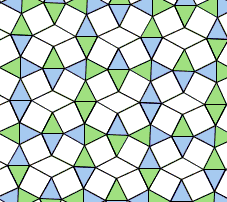# A Semi-regular Tessellation on Hinges C

The applet implements a hinged realization of one [Steinhaus, #82] semi-regular plane tessellations. The tessellation itself is identified as (4, 3, 3, 4, 3) because 5 regular polygons meet at every vertex: a square, followed by two equilateral triangles, followed by a square and then again by an equilateral triangle. In particular this is what makes it semi-regular: a semi-regular tessellation combines more than one kind of regular polygons, but the same arrangement at every vertex.There are two ways to set this tessellation on hinges. Something has to give. We may only preserve either the squares or the equilateral triangles, but not both. Accordingly, there are possible modifications. The one below lets loose the equilateral triangles so that they are free to transform into parallelograms. The squares are then bound to split into two sizes.

### This applet requires Sun's Java VM 2 which your browser may perceive as a popup. Which it is not. If you want to see the applet work, visit Sun's website at https://www.java.com/en/download/index.jsp, download and install Java VM and enjoy the applet.

 What if applet does not run?

### References

• H. Steinhaus, Mathematical Snapshots, umpteen edition, Dover, 1999
• D. Wells, Hidden connections, double meanings: A mathematical exploration, Cambridge University Press, 1988### Plane Tessellations

• Dancing Squares or a Hinged Plane Tessellation
• Dancing Rectangles Model Auxetic Behavior
• A Hinged Realization of a Plane Tessellation
• A Semi-regular Tessellation on Hinges A
• A Semi-regular Tessellation on Hinges B
• Escher's Theorem
• Napoleon Theorem by Plane Tessellation
• Parallelogram Law: A Tessellation
• Simple Quadrilaterals Tessellate the Plane
• Pythagorean Theorem By Plane Tessellation
• Pythagorean Theorem a la Friedrichs
• Pythagorean Theorem By Hexagonal Tessellation
• Hinged Greek Cross Tessellation
• Pythagorean Theorem: A Variant of Proof by Tessellation
•Copyright © 1996-2018 Alexander Bogomolny

•  64842753